# NR Cell Search and MIB and SIB1 Recovery

This example demonstrates how to use 5G Toolbox™ to synchronize, demodulate, and decode a live gNodeB signal. The example decodes the master information block (MIB) and the first of the system information blocks (SIB1). Decoding MIB and SIB1 requires a comprehensive receiver, capable of demodulating and decoding the majority of the downlink channels and signals.

### Introduction

Before user equipment (UE) can communicate with the network, it must perform cell search and selection procedures and obtain initial system information. The first steps in that process are acquiring frame synchronization, finding out the cell identity and decoding the MIB and SIB1. This example shows how to perform these steps by using 5G Toolbox.

You can use this example with a captured waveform of I/Q samples or generate a local waveform containing a synchronization signal (SS) burst and SIB1 using nrWaveformGenerator. For locally generated waveforms, the example performs these steps:

• Waveform generation: Configure and generate a synchronization signal burst carrying the MIB, CORESET 0, PDCCH and PDSCH carrying SIB1 by using the downlink waveform generator from 5G Toolbox. The transmitter can enhance the SNR of one SS block, but it does not perform beamforming. For more information on SSB beamforming, see NR SSB Beam Sweeping.

• AWGN: Apply additive white Gaussian noise (AWGN) to the waveform.

• Receiver: Apply various synchronization and demodulation processes to the received waveform to establish the system frame number, cell identity and SSB, and decode the MIB. These provide the information required for blind decoding of downlink control information (DCI) in a PDCCH. The receiver uses DCI to configure the PDSCH demodulator, decode DL-SCH and finally recover the SIB1.

These figures show the processing steps inside the receiver.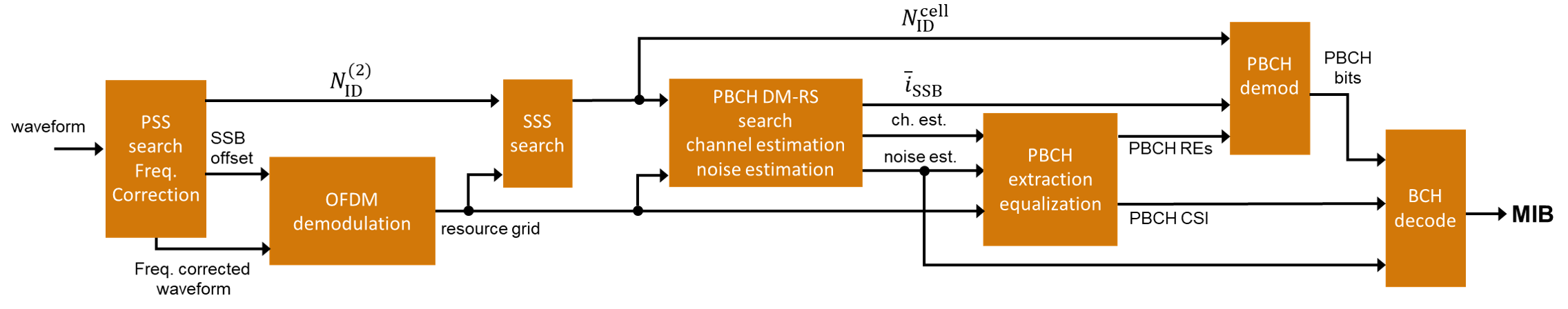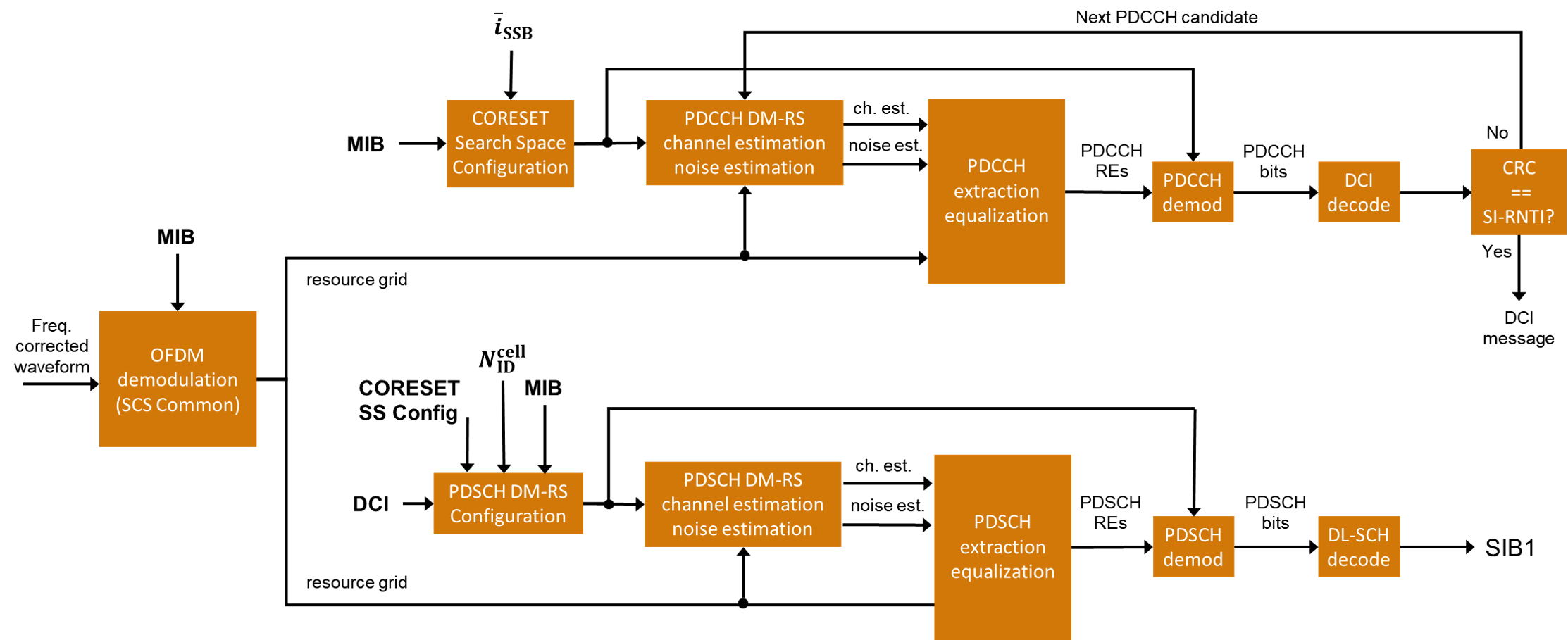To synchronize and demodulate the received waveform, this information is needed:

• The waveform sample rate to demodulate the received waveform.

• The carrier center frequency to apply symbol phase compensation to the received waveform.

• The minimum channel bandwidth to determine CORESET 0 frequency resources. TS 38.101-1 Table 5.3.5-1 [ 1 ] describes the channel bandwidths for each NR band.

• The SS block pattern (Case A...E) to determine the subcarrier spacing of the SS/PBCH blocks. UE searches for SS block patterns based on the NR operating band. For more information, see TS 38.104 Tables 5.4.3.3-1 and 5.4.3.3-2 [ 2 ].

• The number of SS/PBCH blocks in a burst (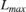) to calculate parameters of the PBCH DM-RS sequences and PBCH descrambling. These parameters depend on the SS/PBCH block index as described in TS 38.211 Sections 7.3.3.1 and 7.4.1.4.1 [ 3 ]. TS 38.213 Section 4.1 [ 5 ] describes the set of SS/PBCH blocks in a burst in each case. UE knows the value ofbased on the SS block pattern and the NR operating band.

loadFromFile = 0; % Set to 1 to load a captured waveform if loadFromFile % Load captured waveform rx = load('capturedWaveformSIB1.mat'); rxWaveform = rx.waveform; % Configure receiver sample rate (samples/second) rxSampleRate = rx.sampleRate; % Symbol phase compensation frequency. Specify the carrier center % frequency or set to 0 to disable symbol phase compensation fPhaseComp = rx.fPhaseComp; % Carrier center frequency (Hz) % Set the minimum channel bandwidth for the NR band required to % configure CORESET 0 in FR1 (See TS 38.101-1 Table 5.3.5-1) minChannelBW = rx.minChannelBW; % 5, 10, 40 MHz % Configure necessary burst parameters at the receiver. The SSB pattern % can be 'Case A','Case B','Case C' for FR1 or 'Case D','Case E' for % FR2. The maximum number of blocks L_max can be 4 or 8 for FR1 and 64 % for FR2. refBurst.BlockPattern = rx.ssbBlockPattern; refBurst.L_max = rx.L_max; else % Generate waveform containing SS burst and SIB1 % Configure the cell identity config = struct(); config.NCellID = 102; % Configure an SS burst config.BlockPattern = 'Case B'; % FR1: 'Case A','Case B','Case C'. FR2: 'Case D','Case E' config.TransmittedBlocks = ones(1,8); % Bitmap of SS blocks transmitted config.SubcarrierSpacingCommon = 15; % SIB1 subcarrier spacing in kHz (15 or 30 for FR1. 60 or 120 for FR2) config.EnableSIB1 = 1; % Set to 0 to disable SIB1 % Set the minimum channel bandwidth for the NR band required to % configure CORESET0 in FR1 (See TS 38.101-1 Table 5.3.5-1) config.MinChannelBW = 5; % 5, 10, 40 MHz % Configure and generate a waveform containing an SS burst and SIB1 wavegenConfig = hSIB1WaveformConfiguration(config); [txWaveform,waveInfo] = nrWaveformGenerator(wavegenConfig); txOfdmInfo = waveInfo.ResourceGrids(1).Info; % Introduce a beamforming gain by boosting the SNR of one SSB and % associated SIB1 PDCCH and PDSCH ssbIdx = 0; % Index of the SSB to boost (0-based) boost = 6; % SNR boost in dB txWaveform = hSIB1Boost(txWaveform,wavegenConfig,waveInfo,ssbIdx,boost); % Add white Gaussian noise to the waveform rng('default'); % Reset the random number generator SNRdB = 20; % SNR for AWGN rxWaveform = awgn(txWaveform,SNRdB-boost,-10*log10(double(txOfdmInfo.Nfft))); % Configure receiver % Sample rate rxSampleRate = txOfdmInfo.SampleRate; % Symbol phase compensation frequency (Hz). The function % nrWaveformGenerator does not apply symbol phase compensation to the % generated waveform. fPhaseComp = 0; % Carrier center frequency (Hz) % Minimum channel bandwidth (MHz) minChannelBW = config.MinChannelBW; % Configure necessary burst parameters at the receiver refBurst.BlockPattern = config.BlockPattern; refBurst.L_max = numel(config.TransmittedBlocks); end % Get OFDM information from configured burst and receiver parameters nrbSSB = 20; scsSSB = hSSBurstSubcarrierSpacing(refBurst.BlockPattern); rxOfdmInfo = nrOFDMInfo(nrbSSB,scsSSB,'SampleRate',rxSampleRate); % Display spectrogram of received waveform figure; nfft = rxOfdmInfo.Nfft; spectrogram(rxWaveform(:,1),ones(nfft,1),0,nfft,'centered',rxSampleRate,'yaxis','MinThreshold',-130); title('Spectrogram of the Received Waveform')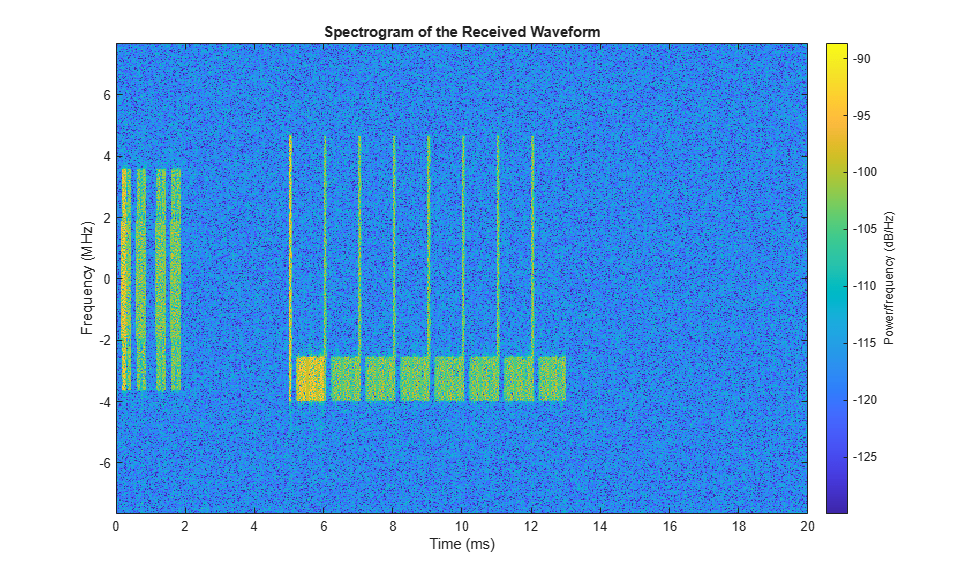### PSS Search and Frequency Offset Correction

The receiver performs PSS search and coarse frequency offset estimation following these steps:

• Frequency shift the received waveform with a candidate frequency offset. Candidate offsets are spaced half subcarrier apart. Use searchBW to control the frequency offset search bandwidth.

• Correlate the frequency-shifted received waveform with each of the three possible PSS sequences (NID2) and extract the strongest correlation peak. The reference PSS sequences are centered in frequency. Therefore, the strongest correlation peak provides a measure of coarse frequency offset with respect to the center frequency of the carrier. The peak also indicates which of the three PSS (NID2) has been detected in the received waveform and the time instant of the best channel conditions.

• Estimate frequency offsets below half subcarrier by correlating the cyclic prefix of each OFDM symbol in the SSB with the corresponding useful parts of the OFDM symbols. The phase of this correlation is proportional to the frequency offset in the waveform.

disp(' -- Frequency correction and timing estimation --') % Specify the frequency offset search bandwidth in kHz searchBW = 6*scsSSB; [rxWaveform,freqOffset,NID2] = hSSBurstFrequencyCorrect(rxWaveform,refBurst.BlockPattern,rxSampleRate,searchBW); disp([' Frequency offset: ' num2str(freqOffset,'%.0f') ' Hz']) 
 -- Frequency correction and timing estimation -- Frequency offset: 65 Hz### Time Synchronization and OFDM Demodulation

The receiver estimates the timing offset to the strongest SS block by using the reference PSS sequence detected in the frequency search process. After frequency offset correction, the receiver can assume that the center frequencies of the reference PSS and received waveform are aligned. Finally, the receiver OFDM demodulates the synchronized waveform and extracts the SS block.

% Create a reference grid for timing estimation using detected PSS. The PSS % is placed in the second OFDM symbol of the reference grid to avoid the % special CP length of the first OFDM symbol. refGrid = zeros([nrbSSB*12 2]); refGrid(nrPSSIndices,2) = nrPSS(NID2); % Second OFDM symbol for correct CP length % Timing estimation. This is the timing offset to the OFDM symbol prior to % the detected SSB due to the content of the reference grid nSlot = 0; timingOffset = nrTimingEstimate(rxWaveform,nrbSSB,scsSSB,nSlot,refGrid,'SampleRate',rxSampleRate); % Synchronization, OFDM demodulation, and extraction of strongest SS block rxGrid = nrOFDMDemodulate(rxWaveform(1+timingOffset:end,:),nrbSSB,scsSSB,nSlot,'SampleRate',rxSampleRate); rxGrid = rxGrid(:,2:5,:); % Display the timing offset in samples. As the symbol lengths are measured % in FFT samples, scale the symbol lengths to account for the receiver % sample rate. srRatio = rxSampleRate/(scsSSB*1e3*rxOfdmInfo.Nfft); firstSymbolLength = rxOfdmInfo.SymbolLengths(1)*srRatio; str = sprintf(' Time offset to synchronization block: %%.0f samples (%%.%.0ff ms) \n',floor(log10(rxSampleRate))-3); fprintf(str,timingOffset+firstSymbolLength,(timingOffset+firstSymbolLength)/rxSampleRate*1e3); 
 Time offset to synchronization block: 2200 samples (0.1432 ms) 

### SSS Search

The receiver extracts the resource elements associated to the SSS from the received grid and correlates them with each possible SSS sequence generated locally. The indices of the strongest PSS and SSS sequences combined give the physical layer cell identity, which is required for PBCH DM-RS and PBCH processing.

% Extract the received SSS symbols from the SS/PBCH block sssIndices = nrSSSIndices; sssRx = nrExtractResources(sssIndices,rxGrid); % Correlate received SSS symbols with each possible SSS sequence sssEst = zeros(1,336); for NID1 = 0:335 ncellid = (3*NID1) + NID2; sssRef = nrSSS(ncellid); sssEst(NID1+1) = sum(abs(mean(sssRx .* conj(sssRef),1)).^2); end % Plot SSS correlations figure; stem(0:335,sssEst,'o'); title('SSS Correlations (Frequency Domain)'); xlabel('$N_{ID}^{(1)}$','Interpreter','latex'); ylabel('Magnitude'); axis([-1 336 0 max(sssEst)*1.1]); % Determine NID1 by finding the strongest correlation NID1 = find(sssEst==max(sssEst)) - 1; % Plot selected NID1 hold on; plot(NID1,max(sssEst),'kx','LineWidth',2,'MarkerSize',8); legend(["correlations" "$N_{ID}^{(1)}$ = " + num2str(NID1)],'Interpreter','latex'); % Form overall cell identity from estimated NID1 and NID2 ncellid = (3*NID1) + NID2; disp([' Cell identity: ' num2str(ncellid)]) 
 Cell identity: 102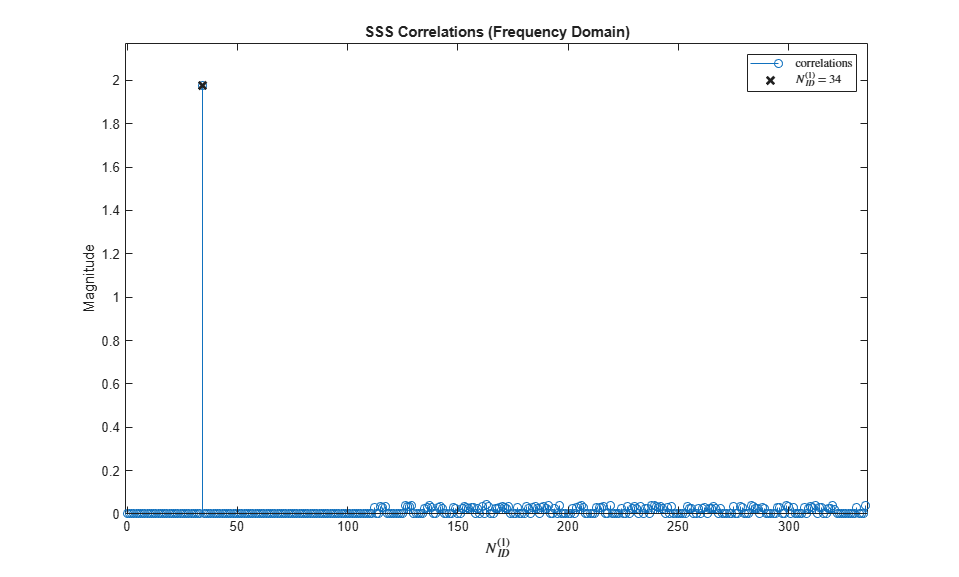### PBCH DM-RS search

In a process similar to SSS search, the receiver constructs each possible PBCH DM-RS sequence and performs channel and noise estimation. The index of the PBCH DM-RS with the best SNR determines the LSBs of the SS/PBCH block index required for PBCH scrambling initialization.

% Calculate PBCH DM-RS indices dmrsIndices = nrPBCHDMRSIndices(ncellid); % Perform channel estimation using DM-RS symbols for each possible DM-RS % sequence and estimate the SNR dmrsEst = zeros(1,8); for ibar_SSB = 0:7 refGrid = zeros([240 4]); refGrid(dmrsIndices) = nrPBCHDMRS(ncellid,ibar_SSB); [hest,nest] = nrChannelEstimate(rxGrid,refGrid,'AveragingWindow',[0 1]); dmrsEst(ibar_SSB+1) = 10*log10(mean(abs(hest(:).^2)) / nest); end % Plot PBCH DM-RS SNRs figure; stem(0:7,dmrsEst,'o'); title('PBCH DM-RS SNR Estimates'); xlabel('$\overline{i}_{SSB}$','Interpreter','latex'); xticks(0:7); ylabel('Estimated SNR (dB)'); axis([-1 8 min(dmrsEst)-1 max(dmrsEst)+1]); % Record ibar_SSB for the highest SNR ibar_SSB = find(dmrsEst==max(dmrsEst)) - 1; % Plot selected ibar_SSB hold on; plot(ibar_SSB,max(dmrsEst),'kx','LineWidth',2,'MarkerSize',8); legend(["SNRs" "$\overline{i}_{SSB}$ = " + num2str(ibar_SSB)],'Interpreter','latex');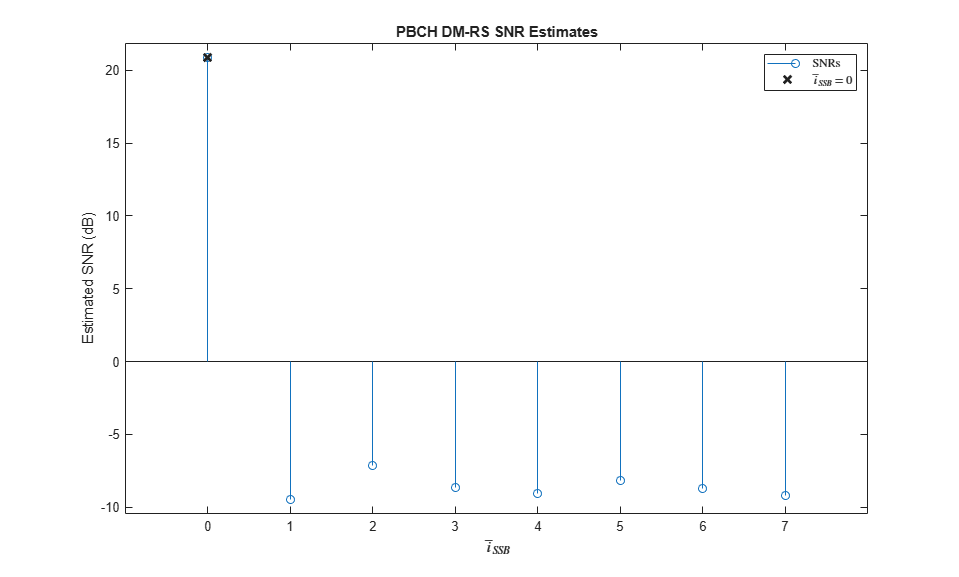### Channel Estimation using PBCH DM-RS and SSS

The receiver estimates the channel for the entire SS/PBCH block using the SSS and PBCH DM-RS detected in previous steps. An estimate of the additive noise on the PBCH DM-RS / SSS is also performed.

refGrid = zeros([nrbSSB*12 4]); refGrid(dmrsIndices) = nrPBCHDMRS(ncellid,ibar_SSB); refGrid(sssIndices) = nrSSS(ncellid); [hest,nest,hestInfo] = nrChannelEstimate(rxGrid,refGrid,'AveragingWindow',[0 1]); 

### PBCH Demodulation

The receiver uses the cell identity to determine and extract the resource elements associated with the PBCH from the received grid. In addition, the receiver uses the channel and noise estimates to perform MMSE equalization. The equalized PBCH symbols are then demodulated and descrambled to give bit estimates for the coded BCH block.

disp(' -- PBCH demodulation and BCH decoding -- ') % Extract the received PBCH symbols from the SS/PBCH block [pbchIndices,pbchIndicesInfo] = nrPBCHIndices(ncellid); pbchRx = nrExtractResources(pbchIndices,rxGrid); % Configure 'v' for PBCH scrambling according to TS 38.211 Section 7.3.3.1 % 'v' is also the 2 LSBs of the SS/PBCH block index for L_max=4, or the 3 % LSBs for L_max=8 or 64. if refBurst.L_max == 4 v = mod(ibar_SSB,4); else v = ibar_SSB; end ssbIndex = v; % PBCH equalization and CSI calculation pbchHest = nrExtractResources(pbchIndices,hest); [pbchEq,csi] = nrEqualizeMMSE(pbchRx,pbchHest,nest); Qm = pbchIndicesInfo.G / pbchIndicesInfo.Gd; csi = repmat(csi.',Qm,1); csi = reshape(csi,[],1); % Plot received PBCH constellation after equalization figure; plot(pbchEq,'o'); xlabel('In-Phase'); ylabel('Quadrature') title('Equalized PBCH Constellation'); m = max(abs([real(pbchEq(:)); imag(pbchEq(:))])) * 1.1; axis([-m m -m m]); % PBCH demodulation pbchBits = nrPBCHDecode(pbchEq,ncellid,v,nest); % Calculate RMS PBCH EVM pbchRef = nrPBCH(pbchBits<0,ncellid,v); evm = comm.EVM; pbchEVMrms = evm(pbchRef,pbchEq); % Display calculated EVM disp([' PBCH RMS EVM: ' num2str(pbchEVMrms,'%0.3f') '%']); 
 -- PBCH demodulation and BCH decoding -- PBCH RMS EVM: 8.687%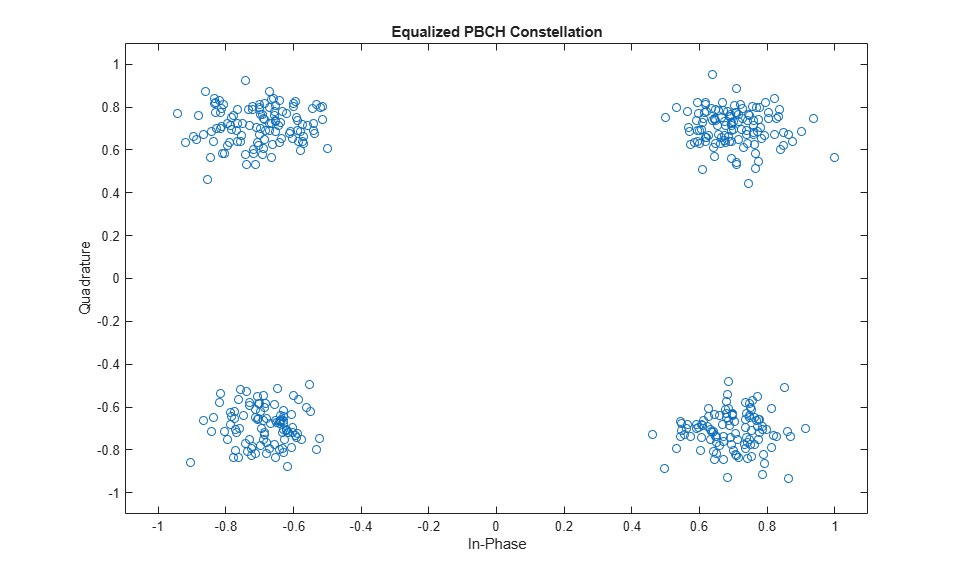### BCH Decoding

The receiver weights BCH bit estimates with channel state information (CSI) from the MMSE equalizer and decodes the BCH. BCH decoding consists of rate recovery, polar decoding, CRC decoding, descrambling, and separating the 24 BCH transport block bits from the 8 additional timing-related payload bits.

% Apply CSI pbchBits = pbchBits .* csi; % Perform BCH decoding including rate recovery, polar decoding, and CRC % decoding. PBCH descrambling and separation of the BCH transport block % bits 'trblk' from 8 additional payload bits A...A+7 is also performed: % A ... A+3: 4 LSBs of system frame number % A+4: half frame number % A+5 ... A+7: for L_max=64, 3 MSBs of the SS/PBCH block index % for L_max=4 or 8, A+5 is the MSB of subcarrier offset k_SSB polarListLength = 8; [~,crcBCH,trblk,sfn4lsb,nHalfFrame,msbidxoffset] = ... nrBCHDecode(pbchBits,polarListLength,refBurst.L_max,ncellid); % Display the BCH CRC disp([' BCH CRC: ' num2str(crcBCH)]); % Stop processing MIB and SIB1 if BCH was received with errors if crcBCH disp(' BCH CRC is not zero.'); return end % Use 'msbidxoffset' value to set bits of 'k_SSB' or 'ssbIndex', depending % on the number of SS/PBCH blocks in the burst if (refBurst.L_max==64) ssbIndex = ssbIndex + (bit2int(msbidxoffset,3) * 8); k_SSB = 0; else k_SSB = msbidxoffset * 16; end % Displaying the SSB index disp([' SSB index: ' num2str(ssbIndex)]); 
 BCH CRC: 0 SSB index: 0 

### MIB and BCH Parsing

The example parses the 24 decoded BCH transport block bits into a MIB message and creates the initialSystemInfo structure with initial system information. This process includes reconstituting the 10-bit system frame number (SFN) NFrame from the 6 MSBs in the MIB and the 4 LSBs in the PBCH payload bits. It also includes incorporating the MSB of the subcarrier offset k_SSB from the PBCH payload bits in the case of L_max=4 or 8 SS/PBCH blocks per burst.

% Parse the last 23 decoded BCH transport block bits into a MIB message. % The BCH transport block 'trblk' is the RRC message BCCH-BCH-Message, % consisting of a leading 0 bit and 23 bits corresponding to the MIB. The % leading bit signals the message type transmitted (MIB or empty sequence). mib = fromBits(MIB,trblk(2:end)); % Do not parse leading bit % Create set of subcarrier spacings signaled by the 7th bit of the decoded % MIB, the set is different for FR1 (L_max=4 or 8) and FR2 (L_max=64) if (refBurst.L_max==64) commonSCSs = [60 120]; else commonSCSs = [15 30]; end initialSystemInfo = struct(); initialSystemInfo.NFrame = mib.systemFrameNumber*2^4 + bit2int(sfn4lsb,4); initialSystemInfo.SubcarrierSpacingCommon = commonSCSs(mib.subCarrierSpacingCommon + 1); initialSystemInfo.k_SSB = k_SSB + mib.ssb_SubcarrierOffset; initialSystemInfo.DMRSTypeAPosition = 2 + mib.dmrs_TypeA_Position; initialSystemInfo.PDCCHConfigSIB1 = info(mib.pdcch_ConfigSIB1); initialSystemInfo.CellBarred = mib.cellBarred; initialSystemInfo.IntraFreqReselection = mib.intraFreqReselection; % Display the MIB structure disp(' BCH/MIB Content:') disp(initialSystemInfo); % Check if a CORESET for Type0-PDCCH common search space (CSS) is present, % according to TS 38.213 Section 4.1 if ~isCORESET0Present(refBurst.BlockPattern,initialSystemInfo.k_SSB) fprintf('CORESET 0 is not present (k_SSB > k_SSB_max).\n'); return end 
 BCH/MIB Content: NFrame: 0 SubcarrierSpacingCommon: 15 k_SSB: 0 DMRSTypeAPosition: 3 PDCCHConfigSIB1: [1x1 struct] CellBarred: 0 IntraFreqReselection: 0 

### OFDM Demodulation on Full Bandwidth

Once the MIB is recovered, the receiver uses common subcarrier spacing and a bandwidth supporting CORESET 0 to OFDM demodulate the frame containing the detected SS block. The receiver determines the CORESET 0 frequency resources in common numerology through an offset from the location of the SSB detected and a bandwidth specified in TS 38.213 Section 13 Tables 13-1 through 13-10 [ 5 ]. The frequency correction process aligned the center of the OFDM resource grid with the center frequency of the SS burst. However, these centers are not necessarily aligned with the center frequency of CORESET 0. This figure shows the relationship between the SSB, CORESET 0 frequency resources and associated PDCCH monitoring occasions.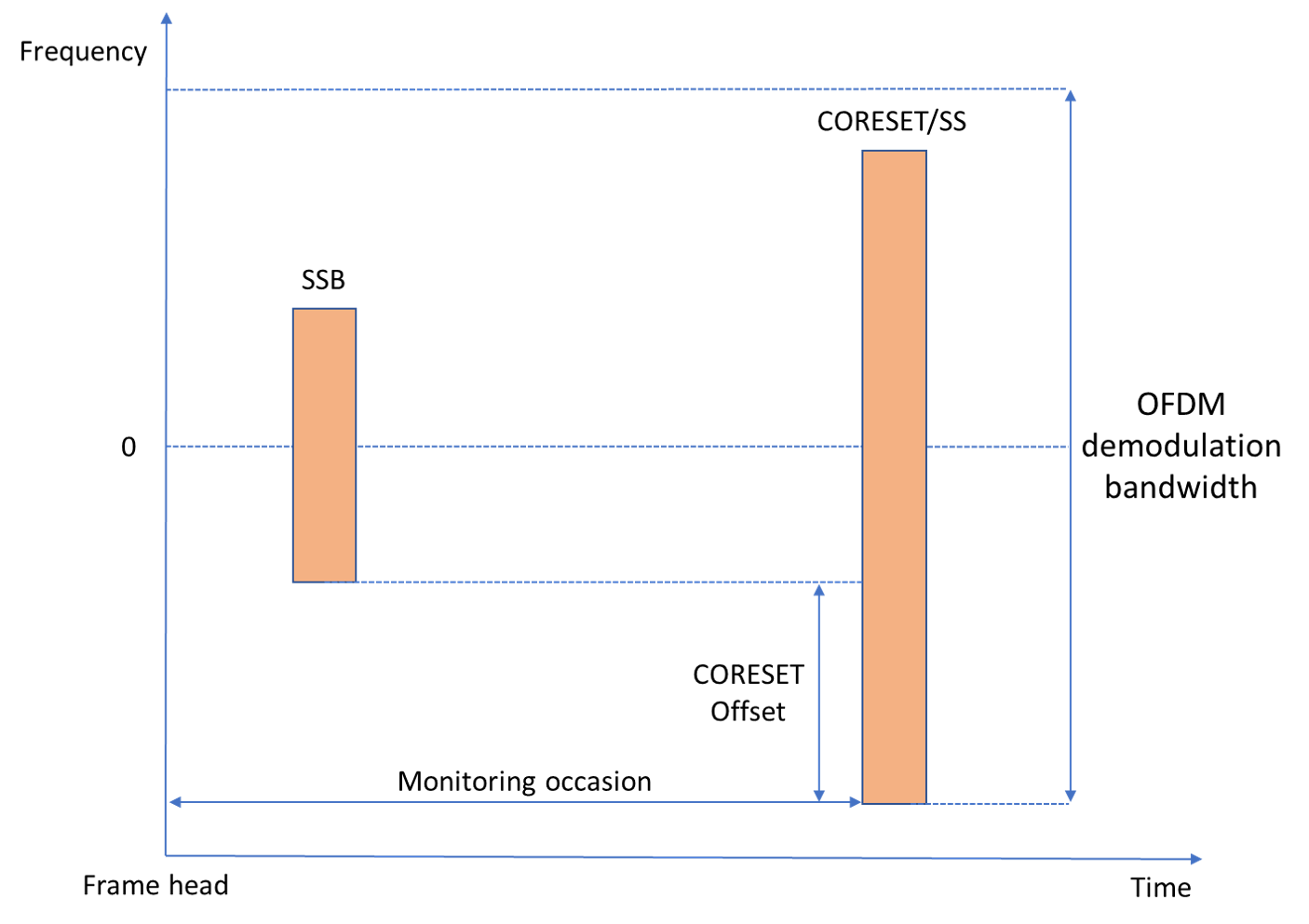Unlike the SS burst, control and data channels must be aligned in frequency with their common resource block (CRB) raster. The value of KSSB indicates the frequency offset of the SSB from that CRB raster. As the frequency correction process centered the SSB in frequency, apply a frequency shift determined by k_SSB to align data and control channels with their CRB before OFDM demodulation

if (refBurst.L_max==64) scsKSSB = initialSystemInfo.SubcarrierSpacingCommon; else scsKSSB = 15; end k_SSB = initialSystemInfo.k_SSB; kFreqShift = k_SSB*scsKSSB*1e3; rxWaveform = rxWaveform.*exp(1i*2*pi*kFreqShift*(0:length(rxWaveform)-1)'/rxSampleRate); % Adjust timing offset to the frame origin frameOffset = hTimingOffsetToFrame(refBurst,timingOffset,ssbIndex,rxSampleRate); % If the frame offset is negative, the frame of interest is incomplete. Add % leading zeros to the waveform to align the wavefom to the frame if frameOffset < 0 rxWaveform = [zeros(-frameOffset,size(rxWaveform,2));rxWaveform]; else rxWaveform = rxWaveform(1+frameOffset:end,:); end % Determine the OFDM demodulation bandwidth using CORESET 0 bandwidth cset0Idx = initialSystemInfo.PDCCHConfigSIB1.controlResourceSetZero; scsCommon = initialSystemInfo.SubcarrierSpacingCommon; scsPair = [scsSSB scsCommon]; [csetNRB,~,csetFreqOffset] = hCORESET0Resources(cset0Idx,scsPair,minChannelBW,k_SSB); % Minimum bandwidth in RB that includes CORESET 0 in received waveform. c0 = csetFreqOffset+10*scsSSB/scsCommon; % CORESET frequency offset from carrier center nrb = 2*max(c0,csetNRB-c0); % Minimum number of RB to cover CORESET 0 if rxSampleRate < nrb*12*scsCommon*1e3 disp(['SIB1 recovery cannot continue. CORESET 0 resources are beyond '... 'the frequency limits of the received waveform for the sampling rate configured.']); return; end % OFDM demodulate received waveform with common subcarrier spacing nSlot = 0; rxGrid = nrOFDMDemodulate(rxWaveform, nrb, scsCommon, nSlot,... 'SampleRate',rxSampleRate,'CarrierFrequency',fPhaseComp); % Display OFDM resource grid and highlight strongest SS block figure; imagesc(abs(rxGrid(:,:,1))); axis xy xlabel('OFDM symbol'); ylabel('Subcarrier'); numFrames = floor(length(rxWaveform)/rxSampleRate/10e-3); sfns = sprintf('(%d...%d)',initialSystemInfo.NFrame, initialSystemInfo.NFrame+numFrames-1); title(['Received Resource Grid. System Frame Number: ' sfns]); highlightSSBlock(refBurst,ssbIndex,nrb,scsPair,kFreqShift)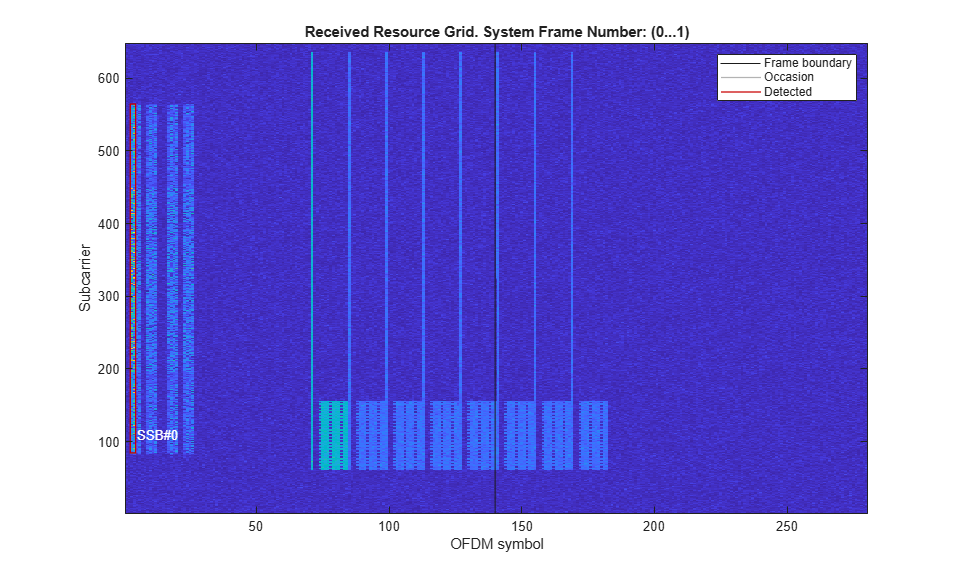### Demodulation of PDCCH and Downlink Control Information Decoding

To blindly search for system information DCI messages in CORESET/SS, the receiver performs these steps:

• Determination of PDCCH monitoring occasions and extraction of the OFDM resource grid containing control information.

• Configuration of CORESET 0, Search spaces and PDCCH.

• Blind search for Format 1_0 DCI messages.

The receiver determines the PDCCH monitoring occasions through a slot and OFDM symbol offset from the location of the SS block detected, as described in TS 38.213 Tables 13-11 and 13-12 [ 5 ] .

[csetNRB,csetDuration,csetOffset,csetPattern] = hCORESET0Resources(cset0Idx,scsPair,minChannelBW,k_SSB); ssIdx = initialSystemInfo.PDCCHConfigSIB1.searchSpaceZero; [ssSlot,ssFirstSym,isOccasion] = hPDCCH0MonitoringOccasions(ssIdx,ssbIndex,scsPair,csetPattern,csetDuration,initialSystemInfo.NFrame); % PDCCH monitoring occasions associated to different SS blocks can be in % different frames. If there are no monitoring occasions in this frame, % there must be one in the next one. slotsPerFrame = 10*scsCommon/15; if ~isOccasion [ssSlot,ssFirstSym,isOccasion] = hPDCCH0MonitoringOccasions(ssIdx,ssbIndex,scsPair,csetPattern,csetDuration,initialSystemInfo.NFrame+1); ssSlot = ssSlot+slotsPerFrame; end % For FR1, UE monitors PDCCH in the Type0-PDCCH CSS over two consecutive % slots for CORESET pattern 1 if csetPattern == 1 monSlotsPerPeriod = 2; else monSlotsPerPeriod = 1; end % Calculate 1-based subscripts of the subcarriers and OFDM symbols for the % slots containing the PDCCH0 associated to the detected SS block in this % and subsequent 2-frame blocks csetSubcarriers = 12*(nrb-20*scsSSB/scsCommon)/2 - csetOffset*12 + (1:csetNRB*12); numRxSym = size(rxGrid,2); symbolsPerSlot = 14; numRxSlots = ceil(numRxSym/symbolsPerSlot); monSlots = ssSlot + (0:monSlotsPerPeriod-1)' + (0:2*slotsPerFrame:(numRxSlots-ssSlot-1)); monSlots = monSlots(:)'; monSymbols = monSlots*symbolsPerSlot + (1:symbolsPerSlot)'; monSymbols = monSymbols(:)'; % Remove monitoring symbols exceeding waveform limits monSymbols(monSymbols > numRxSym) = []; % Check if search space is beyond end of waveform if isempty(monSymbols) disp('Search space slot is beyond end of waveform.'); return; end % Extract slots containing strongest PDCCH from the received grid rxMonSlotGrid = rxGrid(csetSubcarriers,monSymbols,:); 

Configure CORESET, search space, and other PDCCH parameters. CORESET resources and search spaces are configured according to TS 38.213 Section 13 Tables 13-1 through 13-15 [ 5 ]. CCE-to-REG interleaved mapping parameters (REGBundleSize = 6, InterleaverSize = 2, and ShiftIndex = NCellID) are described in TS 38.211 Section 7.3.2.2 [ 3 ]. For CORESET 0, the BWP is the size of the CORESET as described in TS 38.212 Section 7.3.1.0 [ 4 ]. The PDCCH scrambling parameters are nRNTI = 0 and nID = NCellID as described in TS 38.211 Section 7.3.2.3 [ 3 ].

pdcch = hPDCCH0Configuration(ssbIndex,initialSystemInfo,scsPair,ncellid,minChannelBW); % Configure the carrier to span the BWP (CORESET 0) c0Carrier = nrCarrierConfig; c0Carrier.SubcarrierSpacing = initialSystemInfo.SubcarrierSpacingCommon; c0Carrier.NStartGrid = pdcch.NStartBWP; c0Carrier.NSizeGrid = pdcch.NSizeBWP; c0Carrier.NSlot = pdcch.SearchSpace.SlotPeriodAndOffset(2); c0Carrier.NFrame = initialSystemInfo.NFrame; c0Carrier.NCellID = ncellid; 

Search for DCI messages. UE decodes the received PDCCH symbols blindly by monitoring all PDCCH candidates for every aggregation level using the SI-RNTI to identify the right candidate (or instance).

% Specify DCI message with Format 1_0 scrambled with SI-RNTI (TS 38.212 % Section 7.3.1.2.1) dci = DCIFormat1_0_SIRNTI(pdcch.NSizeBWP); disp(' -- Downlink control information message search in PDCCH -- '); siRNTI = 65535; % TS 38.321 Table 7.1-1 dciCRC = true; mSlot = 0; % Loop over all monitoring slots while (mSlot < length(monSlots)) && dciCRC ~= 0 c0Carrier.NSlot = monSlots(mSlot+1); if monSlotsPerPeriod==2 if mod(mSlot,2) pdcch.SearchSpace.SlotPeriodAndOffset(2) = monSlots(2); else pdcch.SearchSpace.SlotPeriodAndOffset(2) = monSlots(1); end end % Get PDCCH candidates according to TS 38.213 Section 10.1 [pdcchInd,pdcchDmrsSym,pdcchDmrsInd] = nrPDCCHSpace(c0Carrier,pdcch); rxSlotGrid = rxMonSlotGrid(:,(1:symbolsPerSlot) + symbolsPerSlot*mSlot,:); rxSlotGrid = rxSlotGrid/max(abs(rxSlotGrid(:))); % Normalization of received RE magnitude % Loop over all supported aggregation levels aLev = 1; while (aLev <= 5) && dciCRC ~= 0 % Loop over all candidates at each aggregation level in SS cIdx = 1; numCandidatesAL = pdcch.SearchSpace.NumCandidates(aLev); while (cIdx <= numCandidatesAL) && dciCRC ~= 0 % Channel estimation using PDCCH DM-RS [hest,nVar,pdcchHestInfo] = nrChannelEstimate(rxSlotGrid,pdcchDmrsInd{aLev}(:,cIdx),pdcchDmrsSym{aLev}(:,cIdx)); % Equalization and demodulation of PDCCH symbols [pdcchRxSym,pdcchHest] = nrExtractResources(pdcchInd{aLev}(:,cIdx),rxSlotGrid,hest); pdcchEqSym = nrEqualizeMMSE(pdcchRxSym,pdcchHest,nVar); dcicw = nrPDCCHDecode(pdcchEqSym,pdcch.DMRSScramblingID,pdcch.RNTI,nVar); % DCI message decoding polarListLength = 8; [dcibits,dciCRC] = nrDCIDecode(dcicw,dci.Width,polarListLength,siRNTI); if dciCRC == 0 disp([' Decoded PDCCH candidate #' num2str(cIdx) ' at aggregation level ' num2str(2^(aLev-1))]) end cIdx = cIdx + 1; end aLev = aLev+1; end mSlot = mSlot+1; end cIdx = cIdx-1; aLev = aLev-1; mSlot = mSlot-1; monSymbols = monSymbols(mSlot*symbolsPerSlot + (1:symbolsPerSlot)); % Calculate RMS PDCCH EVM pdcchRef = nrPDCCH(double(dcicw<0),pdcch.DMRSScramblingID,pdcch.RNTI); evm = comm.EVM; pdcchEVMrms = evm(pdcchRef,pdcchEqSym); % Display calculated EVM disp([' PDCCH RMS EVM: ' num2str(pdcchEVMrms,'%0.3f') '%']); disp([' PDCCH CRC: ' num2str(dciCRC)]); % Highlight CORESET 0/SS corresponding to strongest SSB bounding_box = @(y,x,h,w)rectangle('Position',[x+0.5 y-0.5 w h],'EdgeColor','r'); bounding_box(csetSubcarriers(1),monSymbols(1)+ssFirstSym-1,csetNRB*12,csetDuration); str = sprintf('CORESET 0/SS'); text(monSymbols(1)+ssFirstSym-7,csetSubcarriers(1)-20,0,str,'FontSize',10,'Color','w') if dciCRC disp(' DCI decoding failed.'); return end % Plot received PDCCH constellation after equalization figure; plot(pdcchEqSym,'o'); xlabel('In-Phase'); ylabel('Quadrature') title('Equalized PDCCH Constellation'); m = max(abs([real(pdcchEqSym(:)); imag(pdcchEqSym(:))])) * 1.1; axis([-m m -m m]); % Display the OFDM grid of the slot containing strongest PDCCH figure; imagesc(abs(rxSlotGrid(:,:,1))); axis xy xlabel('OFDM symbol'); ylabel('subcarrier'); title('Slot Containing Strongest PDCCH'); % Highlight PDCCH in resource grid subsPdcch = nrPDCCHSpace(c0Carrier,pdcch,'IndexStyle','Subs'); subsPdcch = double(subsPdcch{aLev}(:,:,cIdx)); x = min(subsPdcch(:,2))-1; X = max(subsPdcch(:,2))-x; y = min(subsPdcch(:,1)); Y = max(subsPdcch(:,1))-y+1; bounding_box(y,x,Y,X); str = sprintf(' PDCCH \n Aggregation Level: %d\n Candidate: %d',2.^(aLev-1),cIdx-1); text(x+X+1,y+Y/2,0,str,'FontSize',10,'Color','w') 
 -- Downlink control information message search in PDCCH -- Decoded PDCCH candidate #1 at aggregation level 8 PDCCH RMS EVM: 10.759% PDCCH CRC: 0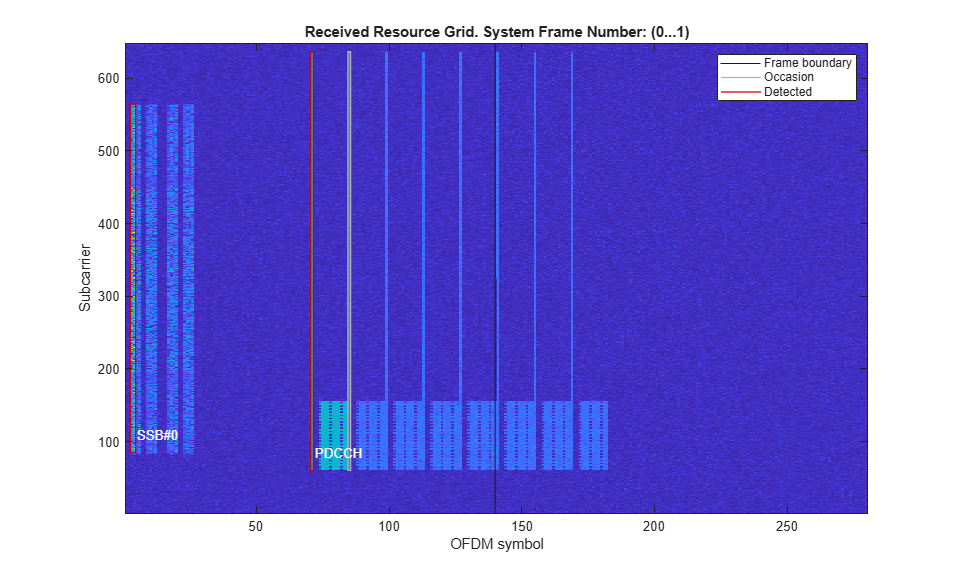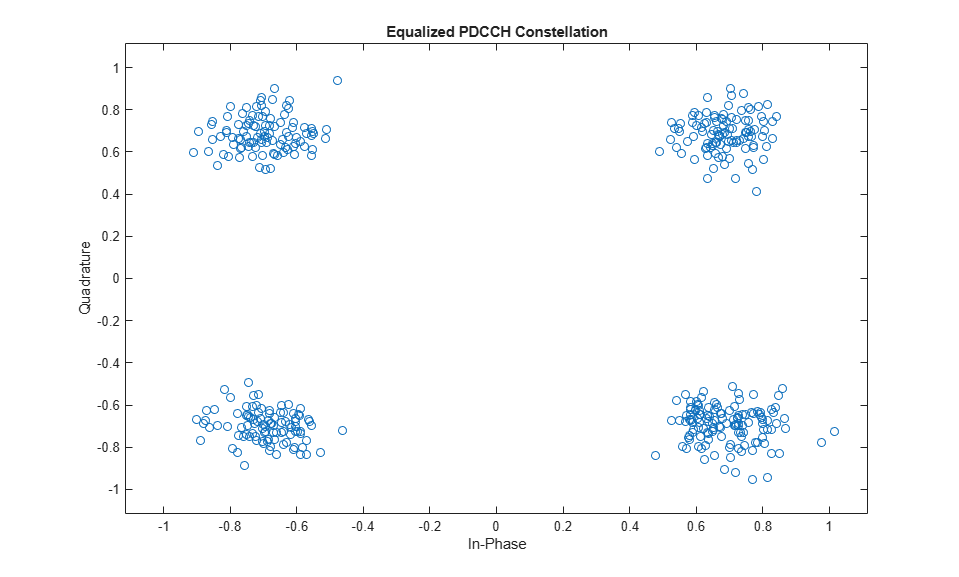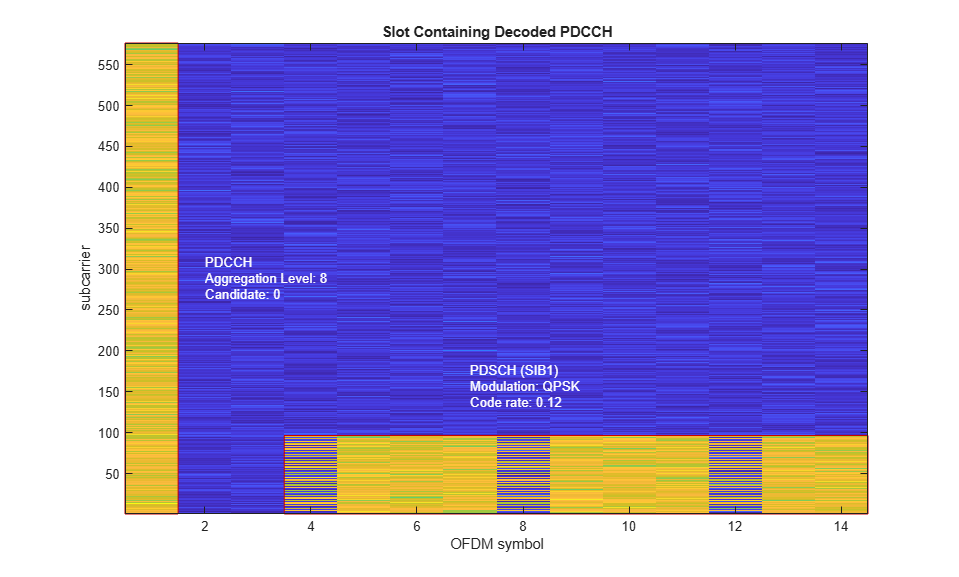### Demodulation of PDSCH, Decoding of DL-SCH and SIB1 Extraction

To recover the first system information block, the receiver performs these steps:

• Determination of PDSCH configuration using cell ID, BCH information, and DCI

• Channel estimation, equalization and demodulation of PDSCH symbols

• Decoding of DL-SCH and SIB1 extraction

disp(' -- PDSCH demodulation and DL-SCH decoding -- ') % Build DCI message structure dci = fromBits(dci,dcibits); % Get PDSCH configuration from cell ID, BCH information, and DCI [pdsch,K_0] = hSIB1PDSCHConfiguration(dci,pdcch.NSizeBWP,initialSystemInfo.DMRSTypeAPosition,csetPattern); % For CORESET pattern 2, the gNodeB can allocate PDSCH in the next slot, % which is indicated by the slot offset K_0 signaled by DCI. For more % information, see TS 38.214 Table 5.1.2.1.1-4. c0Carrier.NSlot = c0Carrier.NSlot+K_0; symbolOffset = symbolsPerSlot*(mSlot+K_0); monSymbols = monSymbols+symbolOffset; rxSlotGrid = rxGrid(csetSubcarriers,monSymbols,:); rxSlotGrid = rxSlotGrid/max(abs(rxSlotGrid(:))); % Normalization of received RE magnitude if K_0 > 0 % Display the OFDM grid of the slot containing associated PDSCH figure; imagesc(abs(rxSlotGrid(:,:,1))); axis xy xlabel('OFDM symbol'); ylabel('subcarrier'); title('Slot Containing PDSCH (Slot Offset K_0 = 1)'); end % PDSCH channel estimation and equalization using PDSCH DM-RS pdschDmrsIndices = nrPDSCHDMRSIndices(c0Carrier,pdsch); pdschDmrsSymbols = nrPDSCHDMRS(c0Carrier,pdsch); 
 -- PDSCH demodulation and DL-SCH decoding -- 

To compensate for the negative effects of a carrier frequency mismatch in symbol phase compensation and channel estimation, the receiver OFDM demodulates the waveform with a set of carrier frequencies over a search bandwidth around fPhaseComp. The search finishes when DL-SCH decoding succeeds or the last frequency has been reached. The minimum search bandwidths that produce equal symbol phase compensation are 1920, 3840, 7680, and 15360 kHz for common subcarrier spacings 15, 30, 60, and 120 kHz, respectively. Increase the search bandwidth up to these values when SIB1 decoding fails and the equalized PDSCH symbols result in a heavily distorted and rotated constellation.

mu = log2(scsCommon/15); bw = 2^mu*100; % Search bandwidth (kHz) freqStep = 2^mu; % Frequency step (kHz) freqSearch = -bw/2:freqStep:bw/2-freqStep; [~,fSearchIdx] = sort(abs(freqSearch)); % Sort frequencies from center freqSearch = freqSearch(fSearchIdx); for fpc = fPhaseComp - kFreqShift + 1e3*freqSearch % OFDM demodulate received waveform nSlot = 0; rxGrid = nrOFDMDemodulate(rxWaveform, nrb, scsCommon, nSlot,... 'SampleRate',rxSampleRate,'CarrierFrequency',fpc); % Extract monitoring slot from the received grid rxSlotGrid = rxGrid(csetSubcarriers,monSymbols,:); rxSlotGrid = rxSlotGrid/max(abs(rxSlotGrid(:))); % Normalization of received RE magnitude % Channel estimation and equalization of PDSCH symbols [hest,nVar,pdschHestInfo] = nrChannelEstimate(rxSlotGrid,pdschDmrsIndices,pdschDmrsSymbols); [pdschIndices,pdschIndicesInfo] = nrPDSCHIndices(c0Carrier,pdsch); [pdschRxSym,pdschHest] = nrExtractResources(pdschIndices,rxSlotGrid,hest); pdschEqSym = nrEqualizeMMSE(pdschRxSym,pdschHest,nVar); % PDSCH demodulation cw = nrPDSCHDecode(c0Carrier,pdsch,pdschEqSym,nVar); % Create and configure DL-SCH decoder with target code rate and % transport block size decodeDLSCH = nrDLSCHDecoder; Xoh_PDSCH = 0; % TS 38.214 Section 5.1.3.2 tcr = hMCS(dci.ModulationCoding); NREPerPRB = pdschIndicesInfo.NREPerPRB; tbsLength = nrTBS(pdsch.Modulation,pdsch.NumLayers,length(pdsch.PRBSet),NREPerPRB,tcr,Xoh_PDSCH); decodeDLSCH.TransportBlockLength = tbsLength; decodeDLSCH.TargetCodeRate = tcr; % Decode DL-SCH [sib1bits,sib1CRC] = decodeDLSCH(cw,pdsch.Modulation,pdsch.NumLayers,dci.RedundancyVersion); if sib1CRC == 0 break; end end % Highlight PDSCH in resource grid subsPdsch = double(nrPDSCHIndices(c0Carrier,pdsch,'IndexStyle','subscript')); x = min(subsPdsch(:,2))-1; X = max(subsPdsch(:,2))-x; y = min(subsPdsch(:,1)); Y = max(subsPdsch(:,1))-y+1; bounding_box(y,x,Y,X); str = sprintf('PDSCH (SIB1) \n Modulation: %s\n Code rate: %.2f',pdsch.Modulation,tcr); text(x+4,y+Y+60,0, str,'FontSize',10,'Color','w') % Plot received PDSCH constellation after equalization figure; plot(pdschEqSym,'o'); xlabel('In-Phase'); ylabel('Quadrature') title('Equalized PDSCH Constellation'); m = max(abs([real(pdschEqSym(:)); imag(pdschEqSym(:))])) * 1.1; axis([-m m -m m]); % Calculate RMS PDSCH EVM, including normalization of PDSCH symbols for any % offset between DM-RS and PDSCH power pdschRef = nrPDSCH(c0Carrier,pdsch,double(cw{1}<0)); evm = comm.EVM; pdschEVMrms = evm(pdschRef,pdschEqSym/sqrt(var(pdschEqSym))); % Display PDSCH EVM and DL-SCH CRC disp([' PDSCH RMS EVM: ' num2str(pdschEVMrms,'%0.3f') '%']); disp([' PDSCH CRC: ' num2str(sib1CRC)]); if sib1CRC == 0 disp(' SIB1 decoding succeeded.'); else disp(' SIB1 decoding failed.'); end 
 PDSCH RMS EVM: 10.835% PDSCH CRC: 0 SIB1 decoding succeeded.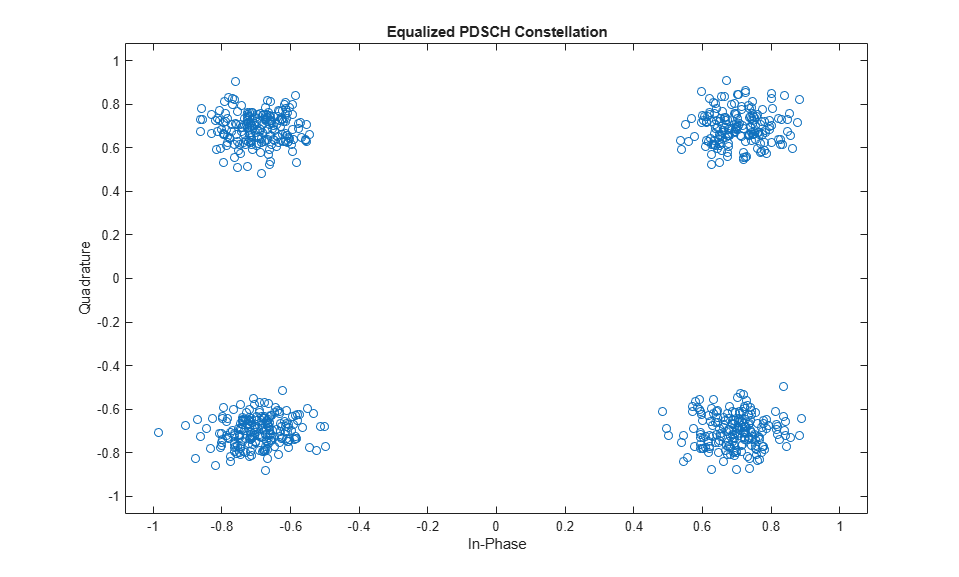### Appendix

This example uses these helper functions:

### References

1. 3GPP TS 38.101-1. "NR; User Equipment (UE) radio transmission and reception; Part 1: Range 1 Standalone" 3rd Generation Partnership Project; Technical Specification Group Radio Access Network.

2. 3GPP TS 38.104. "NR; Base Station (BS) radio transmission and reception." 3rd Generation Partnership Project; Technical Specification Group Radio Access Network.

3. 3GPP TS 38.211. "NR; Physical channels and modulation." 3rd Generation Partnership Project; Technical Specification Group Radio Access Network.

4. 3GPP TS 38.212. "NR; Multiplexing and channel coding." 3rd Generation Partnership Project; Technical Specification Group Radio Access Network.

5. 3GPP TS 38.213. "NR; Physical layer procedures for control." 3rd Generation Partnership Project; Technical Specification Group Radio Access Network.

6. 3GPP TS 38.214. "NR; Physical layer procedures for data." 3rd Generation Partnership Project; Technical Specification Group Radio Access Network.

7. 3GPP TS 38.321. "NR; Medium Access Control (MAC) protocol specification." 3rd Generation Partnership Project; Technical Specification Group Radio Access Network.

### Local functions

function present = isCORESET0Present(ssbBlockPattern,kSSB) switch ssbBlockPattern case {'Case A','Case B','Case C'} % FR1 kssb_max = 23; case {'Case D','Case E'} % FR2 kssb_max = 11; end if (kSSB <= kssb_max) present = true; else present = false; end end function timingOffset = hTimingOffsetToFrame(burst,offset,ssbIdx,rxSampleRate) % As the symbol lengths are measured in FFT samples, scale the symbol % lengths to account for the receiver sample rate. Non-integer delays % are approximated at the end of the process. scs = hSSBurstSubcarrierSpacing(burst.BlockPattern); ofdmInfo = nrOFDMInfo(1,scs,'SampleRate',rxSampleRate); % smallest FFT size for SCS-SR srRatio = rxSampleRate/(scs*1e3*ofdmInfo.Nfft); symbolLengths = ofdmInfo.SymbolLengths*srRatio; % Adjust timing offset to the start of the SS block. This step removes % the extra offset introduced in the reference grid during PSS search, % which contained the PSS in the second OFDM symbol. offset = offset + symbolLengths(1); % Timing offset is adjusted so that the received grid starts at the % frame head i.e. adjust the timing offset for the difference between % the first symbol of the strongest SSB, and the start of the frame burstStartSymbols = hSSBurstStartSymbols(burst.BlockPattern,burst.L_max); % Start symbols in SSB numerology ssbFirstSym = burstStartSymbols(ssbIdx+1); % 0-based % Adjust for whole subframes symbolsPerSubframe = length(symbolLengths); subframeOffset = floor(ssbFirstSym/symbolsPerSubframe); samplesPerSubframe = sum(symbolLengths); timingOffset = offset - (subframeOffset*samplesPerSubframe); % Adjust for remaining OFDM symbols and round offset if not integer symbolOffset = mod(ssbFirstSym,symbolsPerSubframe); timingOffset = round(timingOffset - sum(symbolLengths(1:symbolOffset))); end function highlightSSBlock(refBurst,ssbIndex,commonNRB,scs,kFreqShift) scsSSB = scs(1); scsCommon = scs(2); % Determine frequency origin of the SSB in common numerology bounding_box = @(y,x,h,w)rectangle('Position',[x+0.5 y-0.5 w h],'EdgeColor','r'); scsRatio = scsSSB/scsCommon; ssbFreqOrig = 12*(commonNRB-20*scsRatio)/2+1+kFreqShift/(scsCommon*1e3); % Determine time origin of the SSB in common numerology ssbStartSymbols = hSSBurstStartSymbols(refBurst.BlockPattern,refBurst.L_max); ssbHeadSymbol = ssbStartSymbols(ssbIndex+1)/scsRatio; ssbTailSymbol = floor((ssbStartSymbols(ssbIndex+1)+4)/scsRatio)-1; bounding_box(ssbFreqOrig,ssbHeadSymbol,240*scsRatio,ssbTailSymbol-ssbHeadSymbol+1); str = sprintf('Strongest \n SSB: %d',ssbIndex); text(ssbHeadSymbol,ssbFreqOrig-20,0, str,'FontSize',10,'Color','w') end